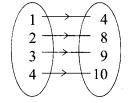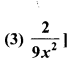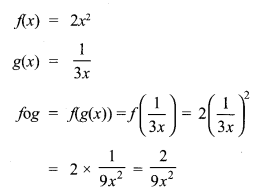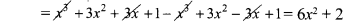# Tamilnadu Board Class 10 Maths Solutions Chapter 1 Relations and Functions Ex 1.6

## Tamilnadu State Board Class 10 Maths Solutions Chapter 1 Relations and Functions Ex 1.6

Question 1.
If n(A × B) = 6 and A = {1, 3} then n(B) is
(1) 1
(2) 2
(3) 3
(4) 6
(3) 3
Hint:
If n(A × B) = 6
A = {1, 1}, n(A) = 2
n(B) = 3

Question 2.
A = {a, b, p}, B = {2, 3}, C = {p, q, r, s} then
n[(A ∪ C) × B] is
(1) 8
(2) 20
(3) 12
(4) 16
(3) 12
Hint:
A = {a, b,p}, B = {2,3}, C = {p, q, r, s}
n (A ∪ C) × B
A ∪C = {a, b,p, q, r,s}
(A ∪C) × B = {{a, 2), (a, 3), (b, 2), (b, 3), (p, 2), (p, 3), (q, 2), (q, 3), (r, 2), (r, 3), (s, 2), (s, 3)
n [(A ∪ C) × B] = 12

Question 3.
If A = {1, 2}, B = {1, 2, 3, 4}, C = {5, 6} and D = {5,6,7,8} then state which of the following statement is true.
(1) (A × C) ⊂ (B × D)
(2) (B × D) ⊂ (A × C)
(3) (A × B) ⊂ (A × D)
(4) (D × A) ⊂ (B × A)
(1) (A × C) ⊂ (B × D)]
Hint:
A = {1, 2}, B = {1, 2, 3, 4},
C = {5, 6}, D ={5,6, 7, 8}
A × C ={(1,5), (1,6), (2, 5), (2, 6)}
B × D = {(1,5),(1,6),(1,7),(1,8),(2,5),(2,6), (2, 7), (2, 8), (3, 5), (3, 6), (3, 7), (3, 8)}
∴ (A × C) ⊂ B × D it is true

Question 4.
If there are 1024 relations from a set A = {1, 2, 3, 4, 5} to a set B, then the number of elements in B is
(1) 3
(2) 2
(3) 4
(4) 6
(2) 2
Hint:
n(A) = 5
n(B) = x
n(A × B) = 1024 = 210
25x = 210
⇒ 5x = 10
⇒ x =2

Question 5.
The range of the relation R = {(x, x2)|x is a prime number less than 13} is
(1) {2,3,5,7}
(2) {2,3,5,7,11}
(3) {4,9,25,49,121}
(4) {1,4,9,25,49,121}
(3) {4, 9, 25, 49,121}]
Hint:
R = {(x, x2)/X is a prime number < 13}
The squares of 2, 3, 5, 7, 11 are
{4, 9, 25,49,121}

Question 6.
If the ordered pairs (a + 2,4) and (5,2a+b)are equal then (a,b) is
(1) (2,-2)
(2) (5,1)
(3) (2,3)
(4) (3,-2)
(4) (3,-2)
Hint:
(a + 2, 4), (5, 2a + b)
a + 2 = 5
a = 3
2a + b = 4
6 + b = 4
b = -2

Question 7.
Let n(A) = m and n(B) = n then the total number of non-empty relations that can be defined from A to B is
(1) mn
(2) nm
(3) 2mn-1
(4) 2mn
(4) 2mn
Hint:
n(A) = m,n(B) = n
n(A × B) = 2mn

Question 8.
If {(a,8),(6,b)}represents an identity function, then the value of a and b are respectively
(1) (8,6)
(2) (8,8)
(3) (6,8)
(4) (6,6)
(1) (8,6)
Hint:
{{a, 8), (6,b)}
a = 8
b = 6

Question 9.
Let A = {1,2,3,4} and B = {4,8,9,10}. A function f : A → B given by f = {(1,4),(2,8),(3,9),(4,10)} is a
(1) Many-one function
(2) Identity function
(3) One-to-one function
(4) Into function
(3) One-to one function
Hint:
A = {1, 2, 3, 4), B = {4, 8, 9,10}Question 10.
If f(x) = 2x2 and g (x) = $$\frac { 1 }{ 3x }$$, Then fog isHint:Queston 11.
If f: A → B is a bijective function and if n(B) = 7, then n(A) is equal to
(1) 7
(2) 49
(3) 1
(4) 14
(1) 7
Hint:
In a bijective function, n(A) = n(B)
⇒ n(A) = 7

Question 12.
Let f and g be two functions given by f = {(0,1), (2,0), (3, -4), (4,2), (5,7)} g = {(0,2), (1, 0), (2, 4), (-4, 2), (7,0)} then the range of fog is
(1) {0,2,3,4,5}
(2) {-4,1,0,2,7}
(3) {1,2,3,4,5}
(4) {0,1,2}
(4) {0,1,2}
Hint:
gof = g(f(x))
fog = f(g(x))
= {(0,2),(1,0),(2,4),(-4,2),(7,0)}
Range of fog = {0,1,2}

Question 13.
Let f(x) = $$\sqrt{1+x^{2}}$$ then
(1) f(xy) = f(x),f(y)
(2) f(xy) > f(x),f(y)
(3) f(xy) < f(x).f(y)
(4) None of these
(3) f(xy) < f(x).f(y)
Hint:Question 14.
If g = {(1, 1),(2, 3),(3, 5),(4, 7)} is a function given by g(x) = ∝x + β then the values of ∝ and β are
(1) (-1,2)
(2) (2,-1)
(3) (-1,-2)
(4) (1,2)
(2) (2,-1)
Hint:
g(x) = αx + β
α = 2
β = -1
g(x) = 2x – 1
g(1) = 2(1) – 1 = 1
g(2) = 2(2) – 1 = 3
g(3) = 2(3) – 1 = 5
g(4) = 2(4) – 1 = 7

Question 15.
f(x) = (x + 1)3 – (x – 1)3 represents a function which is
(1) linear
(2) cubic
(3) reciprocal